# Arithmetic without a Calculator

It is possible to compute the solutions to the numerical problems in this text without the use of a calculator. In fact, depending upon the problem and your ingenuity, accuracy to within a few percent is often achievable. The benefits of doing arithmetic without a calculator are many:

• increased quantitative reasoning skills,
• increased numerical literacy (understanding what the values of numbers really mean), and
• increased reliability (after some practice!).
π ("pi", the exact ratio of the circumference to the diameter of a perfect circle, whose value is approximately 3.1415927, or about 22/7) and

e (the base of the natural logrithms, whose value is approximately 2.7182818, or about 19/7),

every number in physics is a measured quantity, and no measurement is exact. For the purposes of this text, every decimal number can be approximated using relatively simple fractions and their multiples:

fractiondecimal equivalentused to approximate
1/11.0909090909.09
1/10.1.10
1/9.11111111.11
1/8.125.12 or .13
1/7.14285714.14
1/6.16666666.17
1/5.2.20
1/4.25.25
1/3.33333333.33
1/2.5.50

For example, suppose that you need to compute the quotient 381 / 42.7. Your first step is to express these numbers in a modified version of scientific notation:

.381 * 103 / (.427 * 102).
You then notice that
.381 is close to .375, which is 3/8, and that

.427 is almost exactly 3/7.

So you approximate this computation (after dividing out factors of 10) by (3/8 * 10) / (3/7). Further simplifying, our approximate answer is then 7/8 * 10, or 8.75.
Δxabs = | xexact - xapproximate |.
In the example above, the "exact" answer (to 8 digits of accuracy) is 8.9227166. This means that for our approximation, the absolute error was .1727166. We can also define the relative error, which is the absolute error divided by the exact answer, expressed as a percent:
Δxrel = 100 % * Δxabs / xexact.
For our approximation, the relative error was approximately 1.94 %. Since the numerical problems in this text allow up to a 10% error in your answer, this level of accuracy is more than enough for our purposes. The key to this method of computation is to KNOW the fractional equivalents in the table above, and look for opportunities to use them or their multiples. The closer your fractional approximation, the better your results: had we approximated both .381 and .427 as .4, our final answer would have been 10. The relative error in this version of the computation is 12 %, which is not good enough for our purposes!
9.61350, 9.90505, 9.50567, 9.67618, 10.02710, 9.74638, 9.74732, 9.94688.
You did not get the same result each time because there was experimental noise: random variations in the way the experiment was performed each time, as well as small, variable inaccuracies in the equipment combine to introduce variations in the recorded data. It might be meaningful to consider the average of such a data set, but you must compute the experimental uncertainty: a measure of the range of values consistent with the data. For this purpose, we compute the absolute error, using the average as the "exact value". Since the average is 9.77101, the absolute errors in each data point are:
0.15751, 0.13404, 0.26534, 0.09483, 0.25609, 0.02463, 0.02369, 0.17587.
The maximum of these represents the absolute error in the experiment: all data values are then consistent with
9.77101 + or - 0.26534
that is, the range
(9.50567, 10.03635).
The relative error is computed in the same way, using the average as the exact value, to obtain
9.77101 + or - 2.71558 %.
Notice that the absolute error implies that you actually have no significant digits of precision, but the relative error gives a more accurate description of the quality of the results.

### Squares and other Powers

 n n2 1 1 2 4 3 9 4 16 5 25 6 36 7 49 8 64 9 81 10 100

and the approximate square root of two:

21/2 = 1.414 = 10/7

These facts serve as guideposts for your computations. Suppose we need to square 37. Before beginning, it is important to have an intuitive feel for what sort of answer you should get. Since 37 is between 30 and 40, 372 is between 302 and 402:

302 < 372 < 402, or

32 * 102 < 372 < 42 * 102, or

900 < 372 < 1600.

This may seem like a pretty wide margin, but if you get something outside of this range, you know you have made a mistake. This is an example of the sorts of benefits which accrue from doing your own arithmetic: if you make a mistake on your calculator, and do not have any idea of the sort of answer you should end up with, you have no way to know you made a mistake. This is the single most common cause of student errors on tests (other than not studying!).
(a + b)2 = a2 + 2 a * b + b2
and write
372 = (30 + 7)2 = 302 + 2 * 30 * 7 + 72

= 900 + 2 * 210 + 49 = 900 + 420 + 49 = 1369.

Note that this computation was exact: no approximation was involved.

Binomial expansions have other uses in approximation as well. For cubes, use

(a + b)3 = a3 + 3 a2 * b + 3 a * b2 + b3,
and for fourth powers,
(a + b)4 = a4 + 4 a3 * b + 6 a2 * b2 + 4 a * b3 + b4
is helpful. A special use for the latter equation occurs when you have occasion to subtract
x4 - y4,
where x is just a little larger than y. Using the binomial expansion above, we have
x4 - y4
= (y + c)4 - y4

= y4 + 4 y3 * c + 6 y2 * c2 + 4 y * c3 + c4 - y4

= 4 y3 * c + 6 y2 * c2 + 4 y * c3 + c4.

As long as c is much smaller than y, all of the terms after the first are at least an order of magnitude smaller, so
4 y3 * c
becomes an excellent approximation for the original difference.

### Square Roots

b < < a
(read "b is much less than a"), then as an approximation we can ignore the b2 term, and write
(a + b)2 = a2 + 2 a * b.
Now suppose that we need the square root of x, and that we can write x as the product of two numbers which are very close together, differing only by a small number, which we shall call 2b:
x = a * (a + 2b).
Then
x = a2 + 2 a * b

= (a + b)2,

and therefore
x1/2 = a + b.

As an example, suppose we need the square root of 13,421. Before we start, we note that this is 1.3421 * 104, so its square root will be 1.34211/2 * 100, or between 100 and 200. Now it is too hard to factor 13,421, but it is very easy to factor 13,400:

13,400 = 134 * 100.

Using our technique, we write this as

100 * (100 + 34),
so with a = 100 and 34 = 2b, we see that the square root of 13,421 should be close to 117. In fact, 1172 = 13,689, and to 8 digits of accuracy, 13,4211/2 = 115.84904. This means that our relative error in this computation was
100% * | 115.84904 - 117 | / 115.84904 = .993 %
This is very good, but in general your results will be directly related to your ability to find two factors which are very nearly equal. This is more important as the numbers get smaller. As in all of these approximation techniques, practice improves your performance greatly!

Of course, if graphs are available you should make use of them: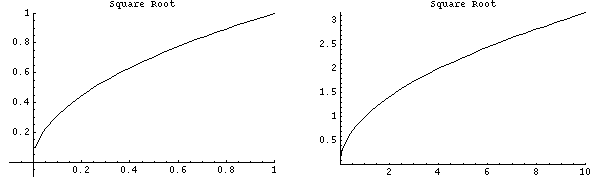### Trigonometry

You can even compute some trigonometric functions without a calculator! As before, however, you need to have some idea of what the answer should be before you begin. We can get such an idea by considering a few right triangles:

from which, with our basic knowledge of the trigonmetric functions, we can build the following table:

 θ sin θ cos θ tan θ 0 0 1 0 30 .5 31/2 / 2 1 / 31/2 37 .6 .8 .75 45 21/2 / 2 21/2 / 2 1 53 .8 .6 4 / 3 60 31/2 / 2 .5 31/2 90 1 0 (infinity)
Note that 21/2/2 is about .70710678 or 7/10, and 31/2 is approximately 1.7320508, so 31/2/2 = .8660254 or about 13/15.

Suppose that you need the sine of 35 degrees. It turns out that within a 10% margin of error,

Sin x = x
for angles from 0 to 42 degrees (about .75 radians). Therefore the sine of 35 degrees is
35 * π / 180
=35 * 22 / (7 * 180)

= 11 / 18

= something between 5/9 (.556) and 6/9 (.667)

= .556 + (.667 - .556) / 2

= .61

The last step is an example of linear interpolation, and is a very useful technique in approximation.

Of course, since

cos θ = sin (π/2 - θ),
this means that the cosine of 55 degrees is also approximately .61 (using our approximation above). If you need the cosine of 35 degrees, you can always use the identity
cos2 θ + sin2 θ = 1
to find that
cos 35 = (1 - (.6 + .01) * .61)1/2
= (1 - .427)1/2

= .5731/2

= 57.31/2 / 10

= .75

(using some of our previous techniques as well). Cos 35 is actually approximately .81915204, so our error is about 8%.

For angles less than 42 degrees, you can also use the approximation

cos θ = 1 - x2 / 2,
which in this example yields
cos 35 = 1 - (11/18)2 / 2
= 1 - 121 / (324 * 2)

= 1 - 121/648

= 1 - 1/5.4

= 1 - (something between 1/5 and 1/6)

= 1 - .18

= .82.

This is of course much better because we did not rely on an approximation for sin 35.

Tangents can be computed using the identity

tan θ = sin θ / cos θ.
In all cases, it is important to know the behaviors of these functions: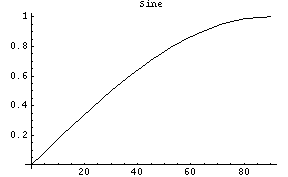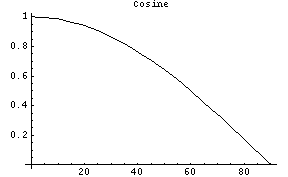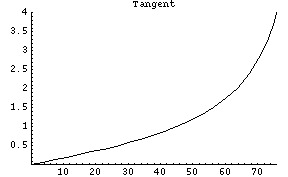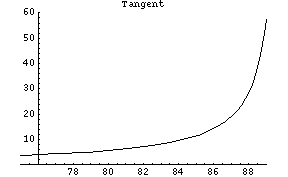sin -θ = - sin θ
cos θ = cos θ
tan -θ = - tan θ
as well as their domains and ranges. The identities for the sum or difference of two angles are also handy:
sin (θ ± φ) = sin (θ) cos (φ) ± cos (θ) sin (φ)
and
cos (θ ± φ) = cos (θ) cos (φ) -+ sin (θ) sin (φ).

Finally, it will be useful to know that for values of x up to .9,

tan-1 x = x - x3 / 3
to within 10% accuracy.

### Error Propagation

It is often necessary to perform computations on measured quantities. The subsequent compounding of error, as well as the process of accounting for it, is called error propagation. For example, consider the following sets of measurements for the variables u and v:

uv
9512
10015
10518

The absolute error in u is 5, and its relative error is 5%; the absolute error in v is 3, and its relative error is 20%. Consider the quantity f = u v2: it can range from 13,680 to 34,020. The relative error in f is then 47%! It is clear that the error has grown considerably.

For our purposes, the root-mean-square (or RMS) error will provide a good way to approximate the propagated error in such a calculation. Suppose that A, B and C are exact quantities (ie., constants) and u and v are measured quantities. If

f1 = A u + B v + C,
the relative error in f1 is
f1rel = √ (A2 urel2 + B2 vrel2).
For
f2 = A u B v C,
the relative error in f2 is
f2 = A √ (B2 urel2 + C2 vrel2).
So the error in a function which is computed from uncertain quantities has a form which depends on the type of computation you are doing. The latter formula (where A is a, B is 1 and C is 2), gives a relative error for our previous example of 40%, commensurate with the 47% error computed by hand.